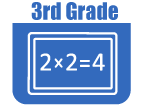IntroductionIn Grade 3, instructional time should focus on four critical areas: (1) developing understanding of multiplication and division and strategies for multiplication and division within 100; (2) developing understanding of fractions, especially unit fractions (fractions with numerator 1); (3) developing understanding of the structure of rectangular arrays and of area; and (4) describing and analyzing two-dimensional shapes.

(1) Students will develop an understanding of the meanings of multiplication and division of whole numbers through activities and problems involving equal-sized groups, arrays, and area models; multiplication is finding an unknown product, and division is finding an unknown factor in these situations. For equal-sized group situations, division can require finding the unknown number of groups or the unknown group size. Students will use properties of operations to calculate products of whole numbers, using increasingly sophisticated strategies based on these properties to solve multiplication and division problems involving single-digit factors. By comparing a variety of solution strategies, students learn the relationship between multiplication and division.

(2) Students will develop an understanding of fractions, beginning with unit fractions. Students will view fractions in general as being built out of unit fractions, and they use fractions along with visual fraction models to represent parts of a whole. Students understand that the size of a fractional part is relative to the size of the whole. For example, 1/2 of the paint in a small bucket could be less paint than 1/3 of the paint in a larger bucket, but 1/3 of a ribbon is longer than 1/5 of the same ribbon because when the ribbon is divided into 3 equal parts, the parts are longer than when the ribbon is divided into 5 equal parts. Students will be able to use fractions to represent numbers equal to, less than, and greater than one. They will solve problems that involve comparing fractions by using visual fraction models and strategies based on noticing equal numerators or denominators.

(3) Students will recognize area as an attribute of two-dimensional regions. They will measure the area of a shape by finding the total number of same-size units of area required to cover the shape without gaps or overlaps, a square with sides of unit length being the standard unit for measuring area. Students will understand that rectangular arrays can be decomposed into identical rows or into identical columns. By decomposing rectangles into rectangular arrays of squares, students will connect area to multiplication and justify using multiplication to determine the area of a rectangle.

(4) Students will describe, analyze, and compare properties of two-dimensional shapes. They will compare and classify shapes by their sides and angles, and connect these with definitions of shapes. Students will also relate their fraction work to geometry by expressing the area of part of a shape as a unit fraction of the whole.The Online Core Resource pages are a collaborative project between the Utah State Board of Education and the Utah Education Network. If you would like to recommend a high quality resource, contact Trish French (Elementary) or Lindsey Henderson (Secondary). If you find inaccuracies or broken links contact resources@uen.org.